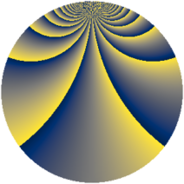# Properties

 Label 1045.2.crLevel $1045$ Weight $2$ Character orbit 1045.cr Rep. character $\chi_{1045}(3,\cdot)$ Character field $\Q(\zeta_{180})$ Dimension $5568$ Sturm bound $240$

# Related objects

## Defining parameters

 Level: $$N$$ $$=$$ $$1045 = 5 \cdot 11 \cdot 19$$ Weight: $$k$$ $$=$$ $$2$$ Character orbit: $$[\chi]$$ $$=$$ 1045.cr (of order $$180$$ and degree $$48$$) Character conductor: $$\operatorname{cond}(\chi)$$ $$=$$ $$1045$$ Character field: $$\Q(\zeta_{180})$$ Sturm bound: $$240$$

## Dimensions

The following table gives the dimensions of various subspaces of $$M_{2}(1045, [\chi])$$.

Total New Old
Modular forms 5952 5952 0
Cusp forms 5568 5568 0
Eisenstein series 384 384 0

## Trace form

 $$5568 q - 36 q^{2} - 36 q^{3} - 36 q^{5} - 72 q^{6} - 54 q^{8} + O(q^{10})$$ $$5568 q - 36 q^{2} - 36 q^{3} - 36 q^{5} - 72 q^{6} - 54 q^{8} - 96 q^{10} - 48 q^{11} - 144 q^{12} - 36 q^{13} - 36 q^{15} - 72 q^{16} - 36 q^{17} - 252 q^{20} - 192 q^{21} - 36 q^{22} - 96 q^{23} + 36 q^{25} + 36 q^{26} - 54 q^{27} + 12 q^{28} - 18 q^{30} - 108 q^{31} - 84 q^{32} - 150 q^{33} - 36 q^{35} - 72 q^{36} - 204 q^{38} - 96 q^{40} + 36 q^{41} - 108 q^{42} - 48 q^{45} - 108 q^{46} - 84 q^{47} + 108 q^{48} - 342 q^{50} + 72 q^{51} - 60 q^{52} - 90 q^{53} + 162 q^{55} - 228 q^{57} - 216 q^{58} - 180 q^{60} - 72 q^{61} - 60 q^{62} + 48 q^{63} - 144 q^{65} - 192 q^{66} - 336 q^{67} + 54 q^{68} - 132 q^{70} - 72 q^{71} - 144 q^{72} - 72 q^{73} - 264 q^{76} - 288 q^{77} - 468 q^{78} - 192 q^{80} + 24 q^{81} + 120 q^{82} - 30 q^{83} - 228 q^{85} - 72 q^{86} - 48 q^{87} + 108 q^{88} - 180 q^{90} - 156 q^{91} + 108 q^{92} + 84 q^{93} - 84 q^{95} - 912 q^{96} + 12 q^{97} + 204 q^{98} + O(q^{100})$$

## Decomposition of $$S_{2}^{\mathrm{new}}(1045, [\chi])$$ into newform subspaces

The newforms in this space have not yet been added to the LMFDB.